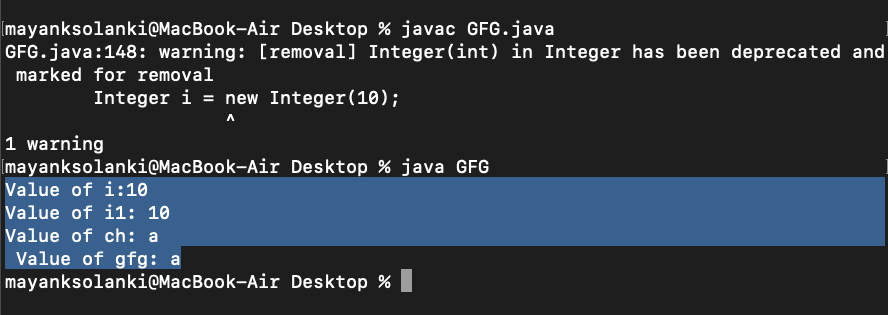Open in App
Not now

# Autoboxing and Unboxing in Java

• Difficulty Level : Easy
• Last Updated : 20 Apr, 2022

In Java, primitive data types are treated differently so do there comes the introduction of wrapper classes where two components play a role namely Autoboxing and Unboxing. Autoboxing refers to the conversion of a primitive value into an object of the corresponding wrapper class is called autoboxing. For example, converting int to Integer class. The Java compiler applies autoboxing when a primitive value is:

• Passed as a parameter to a method that expects an object of the corresponding wrapper class.
• Assigned to a variable of the corresponding wrapper class.

Unboxing on the other hand refers to converting an object of a wrapper type to its corresponding primitive value. For example conversion of Integer to int. The Java compiler applies to unbox when an object of a wrapper class is:

• Passed as a parameter to a method that expects a value of the corresponding primitive type.
• Assigned to a variable of the corresponding primitive type.

The following table lists the primitive types and their corresponding wrapper classes, which are used by the Java compiler for autoboxing and unboxing. Now let us discuss a few advantages of autoboxing and unboxing in order to get why we are using it.

• Autoboxing and unboxing lets developers write cleaner code, making it easier to read.
• The technique lets us use primitive types and Wrapper class objects interchangeably and we do not need to perform any typecasting explicitly.

Example 1:

## Java

 `// Java program to illustrate the Concept` `// of Autoboxing and Unboxing`   `// Importing required classes` `import` `java.io.*;`   `// Main class` `class` `GFG {`   `    ``// Main driver method` `    ``public` `static` `void` `main(String[] args)` `    ``{`   `        ``// Creating an Integer Object` `        ``// with custom value say it be 10` `        ``Integer i = ``new` `Integer(``10``);`   `        ``// Unboxing the Object` `        ``int` `i1 = i;`   `        ``// Print statements` `        ``System.out.println(``"Value of i:"` `+ i);` `        ``System.out.println(``"Value of i1: "` `+ i1);`   `        ``// Autoboxing of character` `        ``Character gfg = ``'a'``;`   `        ``// Auto-unboxing of Character` `        ``char` `ch = gfg;`   `        ``// Print statements` `        ``System.out.println(``"Value of ch: "` `+ ch);` `        ``System.out.println(``" Value of gfg: "` `+ gfg);` `    ``}` `}`

Output:Let’s understand how the compiler did autoboxing and unboxing in the example of Collections in Java using generics.

Example 2:

## Java

 `// Java Program to Illustrate Autoboxing`   `// Importing required classes` `import` `java.io.*;` `import` `java.util.*;`   `// Main class` `class` `GFG {`   `    ``// Main driver method` `    ``public` `static` `void` `main(String[] args)` `    ``{`   `        ``// Creating an empty Arraylist of integer type` `        ``ArrayList al = ``new` `ArrayList();`   `        ``// Adding the int primitives type values` `        ``// using add() method` `        ``// Autoboxing` `        ``al.add(``1``);` `        ``al.add(``2``);` `        ``al.add(``24``);`   `        ``// Printing the ArrayList elements` `        ``System.out.println(``"ArrayList: "` `+ al);` `    ``}` `}`

Output

`ArrayList: [1, 2, 24]`

Output explanation:

In the above example, we have created a list of elements of the Integer type. We are adding int primitive type values instead of Integer Object and the code is successfully compiled. It does not generate a compile-time error as the Java compiler creates an Integer wrapper Object from primitive int i and adds it to the list.

Example 3:

## Java

 `// Java Program to Illustrate Autoboxing`   `// Importing required classes` `import` `java.io.*;` `import` `java.util.*;`   `// Main class` `class` `GFG {`   `    ``// Main driver method` `    ``public` `static` `void` `main(String[] args)` `    ``{`   `        ``// Creating an empty ArrayList of integer type` `        ``List list = ``new` `ArrayList();`   `        ``// Adding the int primitives type values by` `        ``//  converting them into Integer wrapper object` `        ``for` `(``int` `i = ``0``; i < ``10``; i++)`   `            ``System.out.println(` `                ``list.add(Integer.valueOf(i)));` `    ``}` `}`

Output

```true
true
true
true
true
true
true
true
true
true```

Another example of auto and unboxing is to find the sum of odd numbers in a list. An important point in the program is that the operators remainder (%) and unary plus (+=) operators do not apply to Integer objects. But still, code compiles successfully because the unboxing of Integer Object to primitive int value is taking place by invoking intValue() method at runtime.

Example 4:

## Java

 `// Java Program to Illustrate Find Sum of Odd Numbers` `// using Autoboxing and Unboxing`   `// Importing required classes` `import` `java.io.*;` `import` `java.util.*;`   `// Main class` `class` `GFG {`   `    ``// Method 1` `    ``// To sum odd numbers` `    ``public` `static` `int` `sumOfOddNumber(List list)` `    ``{`   `        ``// Initially setting sum to zero` `        ``int` `sum = ``0``;`   `        ``for` `(Integer i : list) {`   `            ``// Unboxing of i automatically` `            ``if` `(i % ``2` `!= ``0``)` `                ``sum += i;`   `            ``// Unboxing of i is done automatically` `            ``// using intvalue implicitly` `            ``if` `(i.intValue() % ``2` `!= ``0``)` `                ``sum += i.intValue();` `        ``}`   `        ``// Returning the odd sum` `        ``return` `sum;` `    ``}`   `    ``// Method 2` `    ``// Main driver method` `    ``public` `static` `void` `main(String[] args)` `    ``{`   `        ``// Creating an empty ArrayList of integer type` `        ``List list = ``new` `ArrayList();`   `        ``// Adding the int primitives type values to List` `        ``for` `(``int` `i = ``0``; i < ``10``; i++)` `            ``list.add(i);`   `        ``// Getting sum of all odd numbers in List` `        ``int` `sumOdd = sumOfOddNumber(list);`   `        ``// Printing sum of odd numbers` `        ``System.out.println(``"Sum of odd numbers = "` `                           ``+ sumOdd);` `    ``}` `}`

Output

`Sum of odd numbers = 50`